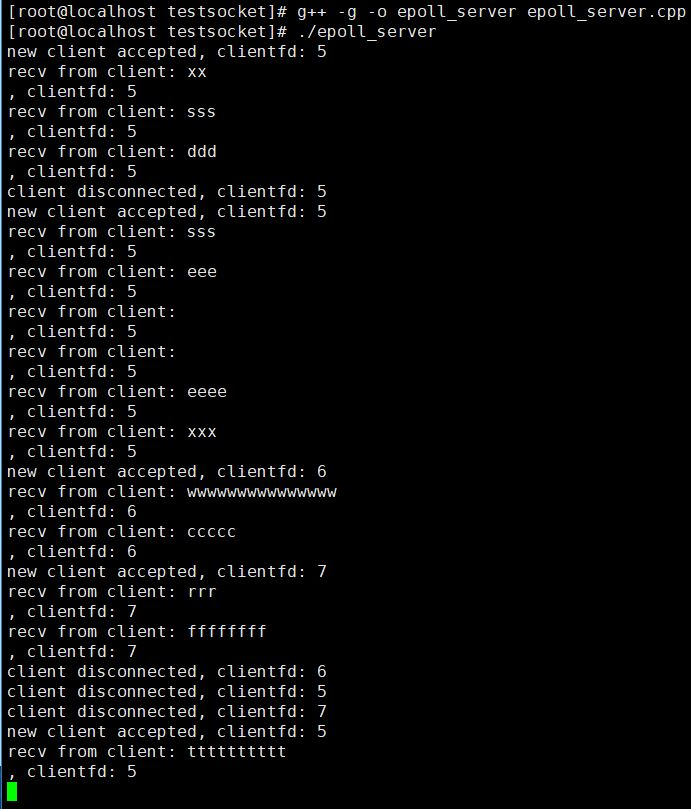• 2021-01-11
• 浏览 (364)

# Linux epoll 模型（含LT 模式和 ET 模式详解）

``````#include <sys/epoll.h>

int epoll_create(int size);
``````

``````int epoll_ctl(int epfd, int op, int fd, struct epoll_event* event);
``````

• 参数 epfd 即上文提到的 epollfd；

• 参数 op，操作类型，取值有 EPOLL_CTL_ADDEPOLL_CTL_MODEPOLL_CTL_DEL，分别表示向 epollfd 上添加、修改和移除一个其他 fd，当取值是 EPOLL_CTL_DEL，第四个参数 event 忽略不计，可以设置为 NULL；

• 参数 fd，即需要被操作的 fd；

• 参数 event，这是一个 epoll_event 结构体的地址，epoll_event 结构体定义如下：

``````  struct epoll_event
{
uint32_t     events;      /* 需要检测的 fd 事件，取值与 poll 函数一样 */
epoll_data_t data;        /* 用户自定义数据 */
};
``````

epoll_event 结构体的 data 字段的类型是 epoll_data_t，我们可以利用这个字段设置一个自己的自定义数据，它本质上是一个 Union 对象，在 64 位操作系统中其大小是 8 字节，其定义如下：

``````  typedef union epoll_data
{
void*         ptr;
int          fd;
uint32_t     u32;
uint64_t     u64;
} epoll_data_t;
``````
• 函数返回值epoll_ctl 调用成功返回 0，调用失败返回 -1，你可以通过 errno 错误码获取具体的错误原因。

``````int epoll_wait(int epfd, struct epoll_event* events, int maxevents, int timeout);
``````

epoll_wait 调用成功会返回有事件的 fd 数目；如果返回 0 表示超时；调用失败返回 -1。

epoll_wait 使用示例如下：

``````while (true)
{
epoll_event epoll_events;
int n = epoll_wait(epollfd, epoll_events, 1024, 1000);
if (n < 0)
{
//被信号中断
if (errno == EINTR)
continue;

//出错，退出
break;
}
else if (n == 0)
{
//超时，继续
continue;
}

for (size_t i = 0; i < n; ++i)
{
// 处理可读事件
if (epoll_events[i].events & POLLIN)
{
}
// 处理可写事件
else if (epoll_events[i].events & POLLOUT)
{
}
//处理出错事件
else if (epoll_events[i].events & POLLERR)
{
}
}
}
``````

## epoll_wait 与 poll 的区别

epoll_wait 函数调用完之后，我们可以直接在 event 参数中拿到所有有事件就绪的 fd，直接处理即可（event 参数仅仅是个出参）；而 poll 函数的事件集合调用前后数量都未改变，只不过调用前我们通过 pollfd 结构体的 events 字段设置待检测事件，调用后我们需要通过 pollfd 结构体的 revents 字段去检测就绪的事件（ 参数 fds 既是入参也是出参）。

``````1. 你把苹果挨个投入到 epoll 机器中(调用 epoll_ctl);
2. 调用 epoll_wait 加工，你直接通过另外一个袋子就能拿到所有熟苹果。
``````

``````1. 把收到的苹果装入一个袋子里面然后调用 poll 加工；
2. 调用结束后，拿到原来的袋子，袋子中还是原来那么多苹果，只不过熟苹果被贴上了标签纸，你还是需要挨个去查看标签纸挑选熟苹果。
``````

## LT 模式和 ET 模式

• 对于水平触发模式，一个事件只要有，就会一直触发；
• 对于边缘触发模式，只有一个事件从无到有才会触发。

``````1. 低电平 => 高电平
2. 高电平 => 高电平
``````

``````1. 低电平 => 高电平
``````

socket 可读事件水平模式触发条件：

``````1. socket上无数据 => socket上有数据
2. socket上有数据 => socket上有数据
``````

socket 可读事件边缘模式触发条件：

``````1. socket上无数据 => socket上有数据
``````

socket 可写事件水平模式触发条件：

``````1. socket可写   => socket可写
2. socket不可写 => socket可写
``````

socket 可写事件边缘模式触发条件：

``````1. socket不可写 => socket可写
``````

``````bool TcpSession::RecvEtMode()
{
//每次只收取256个字节
char buff;
while (true)
{
int nRecv = ::recv(clientfd_, buff, 256, 0);
if (nRecv == -1)
{
if (errno == EWOULDBLOCK)
return true;
else if (errno == EINTR)
continue;

return false;
}
//对端关闭了socket
else if (nRecv == 0)
return false;

}

return true;
}
``````

``````/**
* 演示 epoll 通信模型，epoll_server.cpp
* zhangyl 2019.03.16
*/
#include <sys/types.h>
#include <sys/socket.h>
#include <arpa/inet.h>
#include <unistd.h>
#include <fcntl.h>
#include <sys/epoll.h>
#include <poll.h>
#include <iostream>
#include <string.h>
#include <vector>
#include <errno.h>

int main(int argc, char* argv[])
{
//创建一个侦听socket
int listenfd = socket(AF_INET, SOCK_STREAM, 0);
if (listenfd == -1)
{
std::cout << "create listen socket error." << std::endl;
return -1;
}

//将侦听socket设置为非阻塞的
int oldSocketFlag = fcntl(listenfd, F_GETFL, 0);
int newSocketFlag = oldSocketFlag | O_NONBLOCK;
if (fcntl(listenfd, F_SETFL,  newSocketFlag) == -1)
{
close(listenfd);
std::cout << "set listenfd to nonblock error." << std::endl;
return -1;
}

//初始化服务器地址
{
std::cout << "bind listen socket error." << std::endl;
close(listenfd);
return -1;
}

//启动侦听
if (listen(listenfd, SOMAXCONN) == -1)
{
std::cout << "listen error." << std::endl;
close(listenfd);
return -1;
}

//复用地址和端口号
int on = 1;
setsockopt(listenfd, SOL_SOCKET, SO_REUSEADDR, (char *)&on, sizeof(on));
setsockopt(listenfd, SOL_SOCKET, SO_REUSEPORT, (char *)&on, sizeof(on));

//创建epollfd
int epollfd = epoll_create(1);
if (epollfd == -1)
{
std::cout << "create epollfd error." << std::endl;
close(listenfd);
return -1;
}

epoll_event listen_fd_event;
listen_fd_event.events = POLLIN;
listen_fd_event.data.fd = listenfd;

//将侦听socket绑定到epollfd上去
if(epoll_ctl(epollfd, EPOLL_CTL_ADD, listenfd, &listen_fd_event) == -1)
{
std::cout << "epoll_ctl error." << std::endl;
close(listenfd);
return -1;
}

int n;
while (true)
{
epoll_event epoll_events;
n = epoll_wait(epollfd, epoll_events, 1024, 1000);
if (n < 0)
{
//被信号中断
if (errno == EINTR)
continue;

//出错，退出
break;
}
else if (n == 0)
{
//超时，继续
continue;
}

for (size_t i = 0; i < n; ++i)
{
// 事件可读
if (epoll_events[i].events & POLLIN)
{
if (epoll_events[i].data.fd == listenfd)
{
//侦听socket，接受新连接
//接受客户端连接, 并加入到fds集合中
if (clientfd != -1)
{
//将客户端socket设置为非阻塞的
int oldSocketFlag = fcntl(clientfd, F_GETFL, 0);
int newSocketFlag = oldSocketFlag | O_NONBLOCK;
if (fcntl(clientfd, F_SETFL,  newSocketFlag) == -1)
{
close(clientfd);
std::cout << "set clientfd to nonblock error." << std::endl;
}
else
{
epoll_event client_fd_event;
client_fd_event.events = POLLIN;
client_fd_event.data.fd = clientfd;
if(epoll_ctl(epollfd, EPOLL_CTL_ADD, clientfd, &client_fd_event) != -1)
{
std::cout << "new client accepted, clientfd: " << clientfd << std::endl;
}
else
{
std::cout << "add client fd to epollfd error." << std::endl;
close(clientfd);
}
}
}
}
else
{
//普通clientfd,收取数据
char buf = { 0 };
int m = recv(epoll_events[i].data.fd, buf, 64, 0);
if (m == 0)
{
//对端关闭了连接，从epollfd上移除clientfd
if(epoll_ctl(epollfd, EPOLL_CTL_DEL, epoll_events[i].data.fd, NULL) != -1)
{
std::cout << "client disconnected, clientfd: " << epoll_events[i].data.fd << std::endl;
}
close(epoll_events[i].data.fd);
}
else if (m < 0)
{
//出错，从epollfd上移除clientfd
if (errno != EWOULDBLOCK && errno != EINTR)
{
if(epoll_ctl(epollfd, EPOLL_CTL_DEL, epoll_events[i].data.fd, NULL) != -1)
{
std::cout << "client disconnected, clientfd: " << epoll_events[i].data.fd << std::endl;
}
close(epoll_events[i].data.fd);
}
}
else
{
//正常收到数据
std::cout << "recv from client: " << buf << ", clientfd: " << epoll_events[i].data.fd << std::endl;
}
}
}
else if (epoll_events[i].events & POLLERR)
{
//TODO: 暂且不处理
}

}// end  outer-for-loop
}// end  while-loop

//关闭侦听socket
//（理论上应该关闭包括所有clientfd在内的fd，但这里只是为了演示问题，就不写额外的代码来处理啦）
close(listenfd);

return 0;
}
``````